## An object of mass 0.400.40 kg, hanging from a spring with a spring constant of 8.08.0 N/m, is set into an up-and-down simple harmonic motion

Question

An object of mass 0.400.40 kg, hanging from a spring with a spring constant of 8.08.0 N/m, is set into an up-and-down simple harmonic motion. What is the magnitude of the acceleration of the object when it is at its maximum displacement of 0.100.10 m

in progress 0
2 months 2021-07-28T16:14:33+00:00 1 Answers 4 views 0

Acceleration will be equal to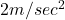Explanation:

We have given mass of the object m = 0.4 kg

Spring constant k = 8 N/m

Maximum displacement of the spring is given x = 0.1 m

From newton’s law force is equal to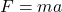…..eqn 1

By hook’s law spring force is equal to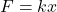…..eqn 2

From equation 1 and equation 2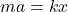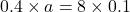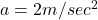So acceleration will be equal to